• likes
• Followers
• Subscribers

Case Report

# Mathematical Analysis of Antibody Rhythms

Bin Zhao1,2, Lichun Liang1

1College of Science, Northwest A&F University, Yangling, Shaanxi, China.

2School of Basic Medical Sciences, Hubei University of Medicine, Shiyan, China.

Corresponding Author: Bin Zhao, College of Science, Northwest Agriculture and Forestry University, Yangling, 712100, China,
Tel: +86 130 28517572; Email: zhaobin835@nwsuaf.edu.cn

Accepted Date: 22 Aug 2016
Published Date: 01 Sep 2016

Citation: Zhao B and Liang L. (2016). Mathematical Analysis of Antibody Rhythms. Mathews J Vet Sci. 1(1): 004.

ABSTRACT

The aim of this study is to develop biomathematical models with important physiological parameters involving immune response for antibody rhythms by using the circadian, infradian and ultradian rhythms of IgY antibody, which could be used in many aspects, for instance to explain the controversies concerning antibody concentration with biological reasons and biomathematical calculations, to optimize the antibody yield in large scale production. In general, this approach could be extended to construct computational models for other antibodies using their biological rhythms to describe their physiological and pathophysiological mechanisms. This approach would aid to utilize the engineered antibodies and their derivatives for various biomedical applications.

KEYWORDS

Antibody Rhythm; Immune Clock; Chronobiology; Biomathematical Model.

INTRODUCTION

The immune system of all living things shows regularly recurring rhythmic variations in numerous frequencies, and the responses of the immune system to an antigen entry could vary in accordance to the chronobiological phenomena. These cyclic oscillations in living things occur for many essential biological processes in order to deal with environmental changes and challenges. Chronobiological phenomena are ubiquitous in living organisms; these periodic (cyclic) episodes are called as biological rhythms (a rhythm is a change that is repeated with a similar pattern). Most of the early reports on biological rhythms focused on circadian rhythms (roughly 24-hours cycle), which could be observed in chicken serum IgY, it has been depicted in mathematics by Forger and Kronauer in 2002 through “van der Pol oscillator”, written in the Lienard phase plane form: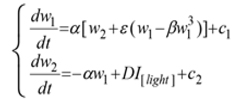where I[light] is an indicator variable, and that is 1 when the light is on, 0 otherwise; D is the change in the derivative of the second state variable induced by the light; c1, c2 are constants.
This treats the state variables as some kind of “stuff”, the second of which is converted to the first, but whose creation is blocked or accelerated by light. In light of, this is not necessarily unrealistic; however it is only a first attempt at modeling changes in circadian rhythms . The further investigations have imposed that, biological cycles having periods shorter or longer than circadian rhythm. For instance, cycles that have periods less than 20-hours are called ultradian rhythms, while cycles that have periods longer than 28-hours are called infradian rhythms. Collectively, these three rhythmic domains comprise a network or web of rhythmic oscillations that in many ways can be linked to the various chemical pathways that perform different functions and occur simultaneously within the same organelle or cell . These cyclic oscillations in living things occur for many essential biological processes in order to deal with environmental changes and challenges.
There is increasing evidence that immune system and its functions are highly and predictably organized in time, for example the immune response to antigen presentation differs both quantitatively and qualitatively according to time . In chrono-immunological study, time dependent variations of immune system parameters are ubiquitous phenomena. Previous study has demonstrated that spleen, lymph nodes, and peritoneal macrophages of mice contain intrinsic circadian clockworks that operate autonomously even ex vivo . Our previous observation demonstrated that FcRn receptor expressed with a regular periodicity in all tested organs . These previous findings explain that, daily rhythms of variety of immunological phenomena and functions are obvious and well known. However, their molecular basis is little understood; thus, it is necessary to elucidate the biological rhythms of immune system (immune clock) to understand the timing mechanism of physiological and pathophysiological immune functions.
Biomathematical or computational models have been proposed in different biological subjects in order to explain various complex phenomena. We have designed this study to demonstrate the rhythmicity of immunoglobulins (synonym for “antibodies” in most situation, refer to a part of the most important molecules in the adaptive immune response) using biomathematical models.
In recent decades, significant and rapid growth of IgY literature shows increasing interest of the scientific community in chicken egg yolk antibody production. IgY antibodies found in birds, reptiles and amphibians are the avian counterpart of IgG. However, IgY antibody production in chicken and poultry has increased due to several advantages over the traditional mammal IgG production. In addition, the unique features of the avian immune system, notably the deposition of antibodies in egg make it ideal for basic immunological research. Recently, we have assessed the chronobiological rhythms of chicken IgY and found significant circaseptan rythms in yolk IgY. Besides the circadian rhythm of IgY is superimposed by an ultradian rhythm (Figure 1 & 2) and the biological reasons behind such oscillations are not clear [6, 7]. The biological oscillations of IgY concentration in serum and yolk IgY were analyzed using the OD450 absorption values (Figure 3). In this study, we constructed some biomathematical models on the basis of IgY oscillations in egg yolk and serum from the view of antibody chronobiological rhythms. Our models could contribute to the efforts regarding to multiple measures of physiology or oscillations in the circadian, infradian and ultradian rhythms of IgY. The following models could be used in many aspects, for instance to address the controversies concerning antibody concentration with biological reasons and biomathematical calculations, to optimize the antibody yield in large scale production.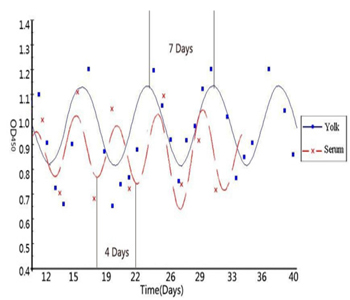Figure 1: Biological rhythm of IgY antibody in serum and Yolk (adopted from He et al., 2014).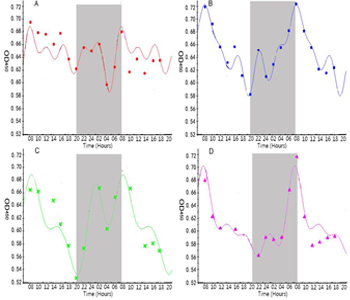Figure 2: Circadian rhythm of IgY antibody in serum (adopted from He et al., 2014).Figure 3: IgY levels in serum and yolk over a 60 day period.

Biomathematical Models and Explanations

Because of the complexity and uncertainty of individuality, the following three biomathematical models have been constructed for the circadian, infradian and ultradian rhythms of IgY in blood and yolk of chickens. Each biomathematical model has two parts namely a rhythm model and a set of transforms of the rhythm model.

###### The circadian rhythm of IgY in blood and yolk of chickens:

On some conditions, such as the reference , the model of circadian rhythm needs us to make a mathematical model to describe by the following two differential equations, which have many aspects, and are full of uncertainty: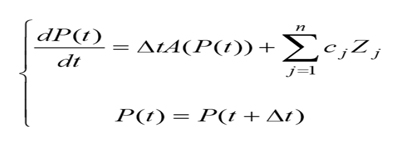where P(t) is the function on circadian rhythm of the time t, cj, = 0 are constants, Zj ( j =1,2,...n.) are the uncertainty of the jth individuality that determine the extent of these n factors related to circadian rhythm, and ?t is the time difference obtained by subtracting universal time.
On the other conditions, the model of circadian rhythm is a simple system of equations, which is described by the following five nonlinear ordinary differential equations :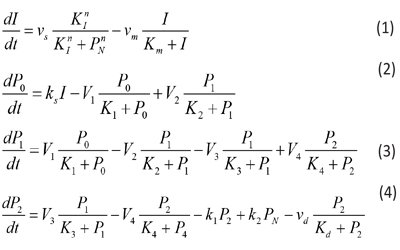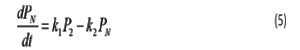where the variables denote the following meanings:
I = IgY levels in serum and yolk
PN =DEV PER (developmental period)
P0 = DEV PER of the ultradian rhythm
P1 = DEV PER of the circadian rhythm P2 = DEV PER of the infradian rhythm
vs = maximum rate of IgY accumulation
vm = maximum rate of IgY degradation
vd = maximum rate of P2 degradation
ks = rate constant characterizing the synthesis of I
k1 and k2 = rate constant characterizing the transportation between P2 and PN
V1 = maximum rate of conversion, P0 ( P1
V2 = maximum rate of reverse conversion, P1 ( P0
V3 = maximum rate of conversion, P1 ( P2
V4 = maximum rate of reverse conversion, P2 ( P1
K1 = Michaelis constant for describing V1
K2 = Michaelis constant for describing V2
K3 = Michaelis constant for describing V3
K4 = Michaelis constant for describing V4
KI = Threshold constant
n = degree of cooperativity
Above model includes a negative feedback between DEV PER and IgY. As DEV PER increases, the concentration of IgY decreases, causing infradian, circadian and ultradian rhythms; but as DEV PER decreases, the concentration of IgY increases, causing DEV PER to increase again and so on, thus result in oscillations [8, 9].
The following values were assigned for the above parameters to produce a circadian rhythm with a period of 24 hours depicting sustainable oscillations simulated:
N=3, vs= 0.76 μM h-1, vm = 0.65 μM h-1, vd = 0.95 μM h-1, ks = 0.38 h-1, k1 = 1.9 h-1, k2 = 1.3 h-1, V1 = 3.2 μM h-1, V2 = 1.58 μM h-1, V3= 5 μM h-1, V4 = 2.5 μM h-1, K1 = K2 = K3 = K4 = 2 μM, Km = 0.5 μM, Kd = 0.2 μM, KI = 1 μM, and n = 4 with initial I, P0, P1, P2, and PNat 0.6 μM, 0.5 μM, 0.5 μM, 0.6 μM, and 1.1 μM, respectively.

For convenience of biomathematical description, the explicit finite difference method has been chosen. The explicit method requires a small time step to ensure the stability and accuracy of the numerical solutions. The code developed here allows the user to set any time step.
For all the simulations here, the default time step is set to 12 min.
As an example, the finite difference of Equation (5) can be written as follows: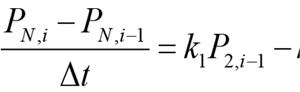In the above equation, the derivative dPN/dt is replaced by the difference quotient,
PN,i - PN,i-1and k1P2 - k2 PN by k1 P2, i-1, - k2 PN, i-1 , such that PN ,i , the concentration of PN at at the current time step i, can be easily calculated from the previous time step. Equations 1, 2, 3, and 4 are solved in a similar manner: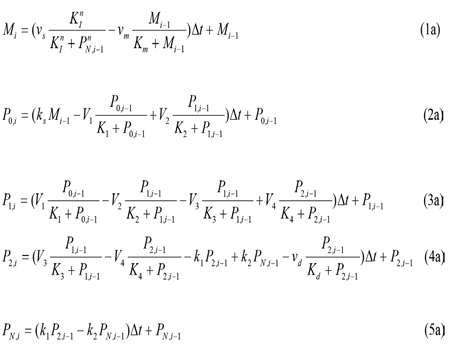With the resultant finite difference equations, the concentration variables M, P0, P1, P2, and PN can now be calculated using iterations from one time step to the next.

The infradian rhythm of IgY in blood and yolk of chickens: To study the infradian rhythm of IgY in blood and yolk of chickens, a “modified coefficient” is added to a selected model parameter, for example, for the above Equation (5a),
PN,i = [(k1+ ampk1 εt) P2,i-1 – (k2 + ampk2εt) PN,i-1] Δt + PN,i-1 where ampk1 and ampk2 are the disturbance amplitudes of the selected parameters k1 and k2, respectively; and εt is the simulation number from 1 to 8. Similar “modified coefficients” are added to all the parameters but M, P0, P1, P2, PN, and Δt in Equations (1a-5a) (e.g. for the parameter V1, the amplitude is ampV1).

The ultradian rhythm of IgY in blood and yolk of chickens: To study the ultradian rhythm of IgY in blood and yolk of chickens, we only needed to assign the value of the simulation number et from 0 to 1.
PN,i = [(k1+ ampk1 et)P2,i-1 – (k2 + ampk2εt)PN,i-1]Δt + PN,i-1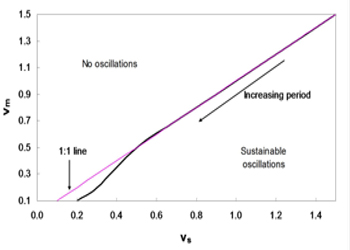Figure 4: Phase diagram of vm and vs. Sustainable oscillations exist only when vs is greater than vm. The domain for such oscillations is relatively large.

As shown in Figure 4, the system with parameters vm and vs below the line produces sustainable oscillations. In brief, a sustainable oscillation requires the rate of accumulation vs to be greater than the rate of degradation vm; otherwise, the IgY concentration would degrade fast rather than accumulation, thereby resulting in the depletion of IgY and a possible collapse of the system. From this assumption, the 1:1 line can be drawn, which roughly coincides with the line obtained from simulations. In addition, the period of the oscillations increase with the decreasing vm and vs.

CONCLUSION

Biological rhythms have been known to play vital roles in organism, and are currently focused as important phenomenon in life science study. Exploring the nature of the clock in immune system and its impact on immune regulations is crucial. In this sense, the biological rhythms of immunoglobulin or antibody have subjected to construct biomathematical models for the current this study. Based on a large number of simulations, it was identified that, there are more sensitive parameters in the circadian model. In the single perturbation cases, we found that the phase shift generally decreases with increasing disturbance amplitude while the recovery time exhibits a nonlinear behavior. In the multiple disturbance cases, the oscillations generally become irregular when the disturbance is approximately greater than 30% of original parameter values. As presented in Figure 5, when the disturbance amplitude and duration of vs are relatively large, the oscillations are irregular. As the duration decreases, the system becomes less sensitive to the disturbance amplitude, as shown in Figure 4. In the parameter space we simulated, the oscillations generally become irregular when the disturbance amplitude for vs exceeds about 30% of the original value of vs, which is 0.76. It is easy to see that vs is one of the most sensitive parameters. We also noticed that when there are three or more consecutive perturbations with the same signs (+/-) and large disturbance amplitudes, the oscillations tend to become irregular (Figure 5)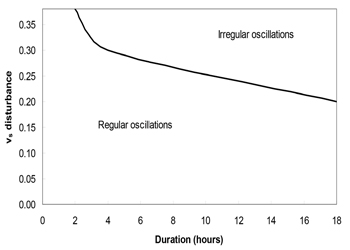Figure 5: Multiple pulse disturbance phase diagram for vs. The oscillations become irregular when the disturbance amplitude of vs exceeds about 30% of its original value.

Despite our work is based on avian IgY antibody, the convenience of chicken-egg model in antibody study has taken into consideration to establish biomathematical models on the basis of our previous IgY rhythm study. Our biomathematical models can be further extended as computational models for the study of chronobiology of other antibodies (eg.: IgG, IgA, IgE and IgM) through their biological rhythms to describe their physiological and/or pathophysiological mechanisms. This would aid to utilize the engineered antibodies and their derivatives for various biomedical applications.

###### CONFLICT OF INTEREST

We have no conflict of interests to disclose and the manuscript has been read and approved by all named authors

Acknowledgments

This work was supported by the Fundamental Research Funds for the Central Universities (2014YB030); Ministry of Education and State Administration of Foreign Experts Affairs “Overseas Teacher” project (MS2011XBNL057); the Key Construction Program (2015SD0018) of International Cooperation Base in S&T, Shaanxi Province, China.

REFERENCES